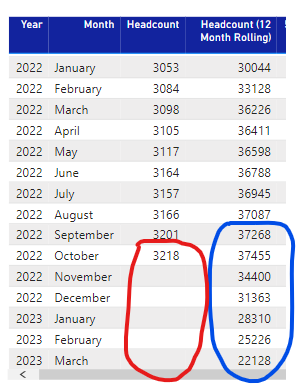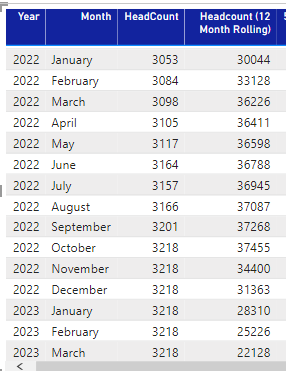cancel
Showing results for
Did you mean:## Add 12 mobile months repeating empty data

Hello friends, good afternoon.

I have the following problem, I need to generate a staff calculation of 12 mobile months projected until the end of the fiscal year (March 2023) but I have data only until October 2022, at the end of November I will have November 2022 and so on.

I have this table:In blue, it is the measure I need but if you realize from noviebre begins to lower the value because it assumes the culmna "headcount" as "0".

What I need is for the red to repeat the last existing value (in this cas 3,218 of October) and so the column of 12 months mobile considers them.

I attach the PBIX in case you can help me, thank you very much!

PBIX

1 ACCEPTED SOLUTION``````Headcount SSOMA=
VAR _SUMMAIRZE =
SUMMARIZE (
'Calendar';
Calendar[Date]. [Year];
Calendar[Date]. [Month];

VAR _CIA = SELECTEDVALUE('Compañía'[EmpresaPivote])
var FilterCia= IF(COUNTROWS(FILTERS('Compañía'[EmpresaPivote]))=1; FALSE(); TRUE())
VAR VoF=IF(FilterCia=FALSE();_CIA; TRUE())
_SUMMAIRZE;
VAR _DATE =
MAXX ( ALL( NominaCons ); NominaCons[Periodo (fecha)] )
VAR _LASTVALUE =
CALCULATE (
[NominaPropios];
all('NominaCons');
'NominaCons'[Periodo (fecha)] = _DATE;
'Compañía'[EmpresaPivote] IN VALUES('Compañía'[EmpresaPivote]);
TipoMineria[Tipo] IN VALUES(TipoMineria[Tipo]);
TipoNomina[TipoNomina] IN VALUES(TipoNomina[TipoNomina]);
)
RETURN
)
RETURN
6 REPLIES 6Helper I

Pleas help me ! , thanks !Community Support

Here I suggest you to try this measure to create a measure.

``````HeadCount =
VAR _SUMMAIRZE =
SUMMARIZE (
Calendar2,
Calendar2[Date].[Year],
Calendar2[Date].[Month],
"HEADCOUNT", CALCULATE ( SUM ( NominaSSOMA[Nomina] ) )
)
_SUMMAIRZE,
VAR _DATE =
MAXX ( ALL ( NominaSSOMA ), NominaSSOMA[Fecha] )
VAR _LASTVALUE =
CALCULATE (
SUM ( NominaSSOMA[Nomina] ),
FILTER ( ALL ( NominaSSOMA ), NominaSSOMA[Fecha] = _DATE )
)
RETURN
)
RETURN

Result is as below.Best Regards,
Rico Zhou

If this post helps, then please consider Accept it as the solution to help the other members find it more quickly.``````Headcount SSOMA=
VAR _SUMMAIRZE =
SUMMARIZE (
'Calendar';
Calendar[Date]. [Year];
Calendar[Date]. [Month];

VAR _CIA = SELECTEDVALUE('Compañía'[EmpresaPivote])
var FilterCia= IF(COUNTROWS(FILTERS('Compañía'[EmpresaPivote]))=1; FALSE(); TRUE())
VAR VoF=IF(FilterCia=FALSE();_CIA; TRUE())
_SUMMAIRZE;
VAR _DATE =
MAXX ( ALL( NominaCons ); NominaCons[Periodo (fecha)] )
VAR _LASTVALUE =
CALCULATE (
[NominaPropios];
all('NominaCons');
'NominaCons'[Periodo (fecha)] = _DATE;
'Compañía'[EmpresaPivote] IN VALUES('Compañía'[EmpresaPivote]);
TipoMineria[Tipo] IN VALUES(TipoMineria[Tipo]);
TipoNomina[TipoNomina] IN VALUES(TipoNomina[TipoNomina]);
)
RETURN
)
RETURNThe solution serves me for the total, but I have a problem when I make a separation by Business Unit

I'll give you the example:If you notice, in the 2nd column the last real value is 479 and that 479 should be repeated, but the total (2629) is repeated and not that of just that business unit

The same in the column of own, should be repeated 750 and repeat 7245. For the total (the sum of both) 1229 should be repeated but 9874 is repeated.I attach the PBIX file to see if you can help me, thank you very much.Super User

Read about LASTNONBLANK and LASTNONBLANKVALUE - they can help you fil these gaps.  NOTE:  they can only do so for measures. You cannot modify column data in DAX.